Atmos. Chem. Phys., 20, 7575–7594, 2020
https://doi.org/10.5194/acp-20-7575-2020
Atmos. Chem. Phys., 20, 7575–7594, 2020
https://doi.org/10.5194/acp-20-7575-2020

Research article 30 Jun 2020

Research article | 30 Jun 2020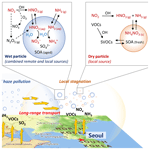# Synergistic enhancement of urban haze by nitrate uptake into transported hygroscopic particles in the Asian continental outflow

Synergistic enhancement of urban haze by nitrate uptake into transported hygroscopic particles in the Asian continental outflow
Jihoon Seo1,2, Yong Bin Lim3, Daeok Youn4, Jin Young Kim1, and Hyoun Cher Jin1 Jihoon Seo et al.
• 1Environment, Health and Welfare Research Center, Korea Institute of Science and Technology, Seoul, 02792, South Korea
• 2School of Earth and Environmental Sciences, Seoul National University, Seoul, 08826, South Korea
• 3Department of Chemical Engineering and Material Science, Ewha Womans University, Seoul, 03760, South Korea
• 4Department of Earth Science Education, Chungbuk National University, Cheongju, 28644, South Korea

Correspondence: Jin Young Kim (jykim@kist.re.kr)

Abstract

Haze pollution is affected by local air pollutants, regional transport of background particles and precursors, atmospheric chemistry related to secondary aerosol formation, and meteorological conditions conducive to physical, dynamical, and chemical processes. In the large, populated and industrialized areas like the Asian continental outflow region, the combination of regional transport and local stagnation often exacerbates urban haze pollution. However, the detailed chemical processes underlying the enhancement of urban haze induced by the combined effect of local emissions and transported remote pollutants are still unclear. Here, we demonstrate an important role of transported hygroscopic particles in increasing local inorganic aerosols, by studying the chemical composition of PM2.5 collected between October 2012 and June 2014 in Seoul, a South Korean megacity in the Asian continental outflow region, using the ISORROPIA II thermodynamic model. PM2.5 measured under the condition of regional transport from the upwind source areas in China was higher in mass concentration and richer in secondary inorganic aerosol (SIA) species (${\mathrm{SO}}_{\mathrm{4}}^{\mathrm{2}-}$, ${\mathrm{NO}}_{\mathrm{3}}^{-}$, and ${\mathrm{NH}}_{\mathrm{4}}^{+}$) and aerosol liquid water (ALW) compared to that measured under non-transport conditions. The secondary inorganic species and ALW were both increased, particularly in cases with high PM2.5 levels, and this indicates inorganic species as a major driver of hygroscopicity. We conclude that the urban haze pollution in a continental outflow region like Seoul, particularly during the cold season, can be exacerbated by ALW in the transported particles, which enhances the nitrate partitioning into the particle phase in NOx- and NH3-rich urban areas. This study reveals the synergistic effect of remote and local sources on urban haze pollution in the downwind region and provides insight into the nonlinearity of domestic and foreign contributions to receptor PM2.5 concentrations in numerical air quality models.

Share
1 Introduction

Fine particulate matter (under 2.5 µm in diameter; PM2.5) in urban areas consists of inorganic species (${\mathrm{SO}}_{\mathrm{4}}^{\mathrm{2}-}$, ${\mathrm{NO}}_{\mathrm{3}}^{-}$, and ${\mathrm{NH}}_{\mathrm{4}}^{+}$) and organic matter (OM) produced by the gas-to-particle conversion of anthropogenic and biogenic precursors (Seinfeld and Pandis, 2016). These particles pose a public health problem due to their adverse effects on the human respiratory and cardiovascular systems (Pope and Dockery, 2006). Consequently, many countries have tried to mitigate urban haze pollution by reducing local precursor emissions (van der A et al., 2017; Kim and Lee, 2018). However, since air pollution is also influenced by transported air pollutants, it is difficult to achieve improved air quality in megacities located in large, populated, and industrialized areas by controlling local emissions (Seo et al., 2018). Synoptic weather conditions cause stagnation and long-range transport that can lead to the accumulation of particles and gaseous precursors from local and remote sources. It can also change local meteorological factors to favorable conditions for secondary aerosol production, such as photo-oxidation and aqueous-phase processing (Sun et al., 2014; Zheng et al., 2015; Seo et al., 2017). Therefore, a better understanding of the combined effects of local emissions, regional transport, and meteorological conditions on urban haze pollution is required to establish appropriate mitigation strategies.

One key aerosol component that affects the formation and growth of haze particles is aerosol liquid water (ALW), which is ubiquitous and abundant in tropospheric fine particles (Nguyen et al., 2016). ALW not only increases the mass of secondary organic aerosols (SOAs) by facilitating the partitioning of gas-phase water-soluble OM into the condensed phase, followed by aqueous-phase reactions (Asa-Awuku et al., 2010; Carlton and Turpin, 2013; McNeill, 2015; Marais et al., 2016), but it also enhances the secondary inorganic aerosol (SIA) mass via nitrate formation through HNO3 uptake and N2O5 hydrolysis (Zhang et al., 2015; Wang et al., 2017) and via sulfate production through the aqueous oxidation of SO2 (Cheng et al., 2016; Wang et al., 2017). Studies of urban haze in the North China Plain reported a simultaneous elevation of the relative humidity (RH), ALW, and SIA, which indicates the hygroscopic properties of inorganic species and the role of ALW in mass transfer into the particles (Liu et al., 2017; Tie et al., 2017; Wu et al., 2018). Combined with the ambient temperature and particle pH, ALW is critical for gas–particle partitioning of inorganic and water-soluble organic acid gases (Guo et al., 2018; Nah et al., 2018). Therefore, regional transport of wet particles to the precursor-rich urban environment will affect haze pollution downwind.

The South Korean capital city of Seoul and its metropolitan area is one of the highly populated megacities in East Asia, with a population of 25 million people, 9 million vehicles, and nearly half of the national gross domestic product, and it has suffered from episodic haze events, particularly during the cold season (Seo et al., 2017, 2018). Although the Seoul metropolitan area is a large anthropogenic emission source of nitrogen oxides (NOx), ammonia (NH3), and volatile organic compounds (VOCs) (NIER, 2018), the effect of transported air pollutants from China cannot be ignored, because of its location downwind from the major emission source region in China like the North China Plain and Yangtze River Delta (Fig. S1 in the Supplement). In particular, severe multiday haze events in the Seoul metropolitan area mostly occur with a specific synoptic pattern, such as an eastward-moving high-pressure system, which induces regional transport of air pollutants from China and, subsequently, local stagnation (Seo et al., 2017, 2018). Therefore, the haze pollution in Seoul becomes severe primarily by the accumulation of local and transported air pollutants. However, a recent numerical modeling study on regional contribution to the particulate concentration in Seoul reported a discrepancy between responses to the reduction in domestic and foreign emissions (Kim et al., 2017), and this implies additional production and growth of local haze particles by nonlinear interactions between local emissions and transported pollutants.

In this study, we explore the combined effects of local and remote sources, ALW and particle pH, and meteorological factors on the formation and growth of urban haze particles, based on daily measurement of PM2.5 chemical compositions in Seoul, backward trajectory analysis, and the ISORROPIA II thermodynamic model (Fountoukis and Nenes, 2007). Different chemical compositions and characteristics of Seoul haze according to the regional transport from China and the local stagnation in the Seoul metropolitan area is investigated from the perspective of inorganic partitioning and water uptake processes. An effective strategy for PM2.5 reduction in Seoul is further discussed using ambient NOx and NH3 levels and analytic calculation of the HNO3${\mathrm{NO}}_{\mathrm{3}}^{-}$ partitioning ratio as a function of ALW, pH, and temperature.

2 Data and methods

## 2.1 Measurements and chemical analysis

Daily PM2.5 sampling was conducted on 210 days between October 2012 and June 2014 at the Korea Institute of Science and Technology (KIST) site in northeastern Seoul (37.603 N, 127.047 E; 58 m above sea level; Fig. S1). PM2.5 samples for determining inorganic and carbonaceous species were collected on 47 mm Teflon filters (Pall Corporation, Port Washington, NY, USA) with a Teflon-coated aluminum cyclone (URG Corporation, Chapel Hill, NC, USA) at a flow rate of 16.7 L min−1 and on 203×254 mm quartz fiber filters (Whatman, Maidstone, UK) with a high-volume air sampler (flow rate of 1000 L min−1; Andersen Instruments, Atlanta, GA, USA), respectively.

The concentrations of inorganic ions (${\mathrm{SO}}_{\mathrm{4}}^{\mathrm{2}-}$, ${\mathrm{NO}}_{\mathrm{3}}^{-}$, Cl, ${\mathrm{NH}}_{\mathrm{4}}^{+}$, K+, Ca2+, Na+, and Mg2+) were measured using a 2000i/SP ion chromatograph (Dionex, Sunnyvale, CA, USA) after sonicating the Teflon filter sample for 30 min in a mixture of 0.5 mL of ethanol and 14.5 mL of distilled deionized water. Using a piece of the quartz fiber filter sample (10×15 mm), the concentrations of organic carbon (OC) and elemental carbon (EC) were measured using a thermal–optical carbon aerosol analyzer (Sunset Laboratory, Tigard, OR, USA) based on National Institute for Occupational Safety and Health (NIOSH) method 5040 (Birch and Cary, 1996). We also identified 17 n-alkanes (C20C36), 15 polycyclic aromatic hydrocarbons (PAHs; C14C24), 19 monocarboxylic acids (C6C20), 19 dicarboxylic acids (C3C11), and 10 sugars (C5C6 and C12) using the extract from one half of the quartz fiber filter sample and a 7890A gas chromatograph (Hewlett Packard, Palo Alto, CA, USA) coupled to a 5975C mass selective detector (Agilent, Santa Clara, CA, USA). The OM∕OC ratios derived from the measured OM and OC concentrations were used to estimate the total OM concentration. The organic compounds identified in this study constitute ∼5 % of the total OM. The analytical procedures used herein are described in detail elsewhere (Seo et al., 2017; Kim et al., 2018). In this study, out of 210 sampling days, 118 daily data that include not only inorganic species but also OM based on the identified organic compounds' information were selected and utilized (Fig. S2).

Note that the PM2.5 sampling on a Teflon filter for inorganic ions was conducted without either a denuder or backup filters, and thus there could be potential sampling artifacts in the results, particularly negative artifacts in semi-volatile ammonium nitrate (Ashbaugh and Eldred, 2004; Chow et al., 2005). Nie et al. (2010) reported that summertime nitrate loss on a Teflon filter from the un-denuded filter sampling without backup filters is ∼75 % at lower nitrate concentrations (<10µg m−3) but only ∼10 % at higher nitrate concentrations (>10µg m−3) due to the formation of particle cake. Considering small evaporative loss in the cold season and the high nitrate concentration in Seoul, we expected small to moderate sampling errors in this study. Sensitivity tests considering potential ammonium nitrate loss from the filter samples show that the assumption of 20 % nitrate loss for the high concentrations with low-temperature groups and 50 % nitrate loss for the low concentrations with moderate-temperature groups does not change our conclusion (Fig. S3).

The hourly concentrations of SO2, NO2, CO, O3, and PM10 at 34 air quality monitoring sites in Seoul (Fig. S1) provided by the Korea Ministry of Environment were averaged over all sites for each day to obtain representative daily concentrations of each species for Seoul (Korea Environment Corporation, 2019). The hourly meteorological data of temperature, RH, wind speed, and solar irradiance at the Seoul weather station (37.571 N, 126.966 E) managed by the Korea Meteorological Administration (KMA) were averaged for each day and used in our analysis (KMA, 2019). Boundary layer height (BLH) was derived from the European Centre for Medium-Range Weather Forecasts Reanalysis Interim (ERA-Interim) data (Dee et al., 2011; http://apps.ecmwf.int/datasets/data/interim-full-daily/, last access: 23 October 2019) at a grid point in Seoul (37.5 N, 127.0 E).

## 2.2 Categorization of measurements

To categorize the daily measurements according to (1) local atmospheric conditions (stagnation or ventilation) and (2) regional impact (transport of pollutants from upwind source area), we used the 72 h backward trajectories from 500 m above the sampling site, obtained with the Hybrid Single-Particle Lagrangian Integrated Trajectory (HYSPLIT) model (https://ready.arl.noaa.gov; Stein et al., 2015) for every hour of each measurement day. We defined a local source area (Seoul metropolitan area) and two major upwind source areas in China (North China Plain and Yangtze River Delta) based on the satellite tropospheric NO2 column density distribution (Fig. 1a) and calculated the average residence time of daily 24 trajectories in the Seoul metropolitan area (tSMA) and the two source areas in China (tCHN). The daily average residence time in each area shows different source characteristics such as the smaller sulfur-to-nitrogen emission ratio in Seoul (∼0.06; 4.5 kt of SOx and 71.1 kt of NOx in 2010; NIER, 2018) compared with that of the Jing–Jin–Ji region in the North China Plain (∼0.71; 2010 kt of SO2 and 2830 kt of NOx in 2010; Li et al., 2017). For example, tCHN is highly correlated not only with particulate ${\mathrm{SO}}_{\mathrm{4}}^{\mathrm{2}-}$ and ${\mathrm{NO}}_{\mathrm{3}}^{-}$ concentrations but also with their precursor (SO2 and NO2) concentrations, while tSMA has statistically significant correlation only with NO2 concentration (Fig. S4).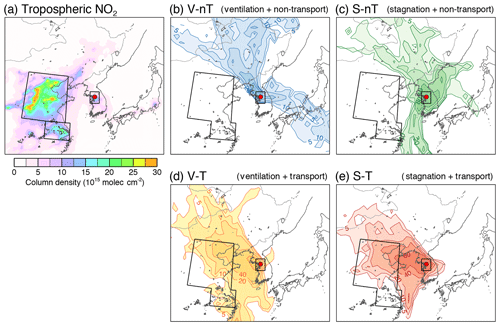Figure 1(a) Tropospheric NO2 column density obtained from the Ozone Monitoring Instrument (OMI) onboard the EOS-Aura satellite, averaged for 2012–2014, and the major anthropogenic emission areas defined in this study (North China Plain, NCP: 33–41 N, 112–121 E; Yangtze River Delta, YRD: 30–33 N, 117–122 E; and Seoul metropolitan area, SMA; 36.5–38.5 N, 126–128 E). (b–e) Trajectory frequency (number of endpoints in each $\mathrm{1}{}^{\circ }\phantom{\rule{0.125em}{0ex}}×\mathrm{1}{}^{\circ }$ grid cell per number of trajectories, %) derived from the HYSPLIT 72 h backward trajectories obtained 500 m above the Korea Institute of Science and Technology (KIST) site and average chemical compositions of particulate matter under 2.5 µm in diameter (PM2.5) for the (b) “local ventilation with no regional transport” (V-nT) group, (c) “local stagnation with no regional transport” (S-nT) group, (d) “local ventilation with regional transport” (V-T) group, and (e) “local stagnation with regional transport” (S-T) group. Seoul is marked with a solid red circle.

Since the medians of both tSMA (for all measurement days) and tCHN (for the measurement days with tCHN≠0 h) were ∼6 h, we applied 6 h as a reference trajectory residence time to categorize daily measurement data into relatively stronger and weaker influences through the local stagnation in the Seoul metropolitan area (with tSMA) and the regional transport from China (with tCHN). The measurement days were classified into one of four groups and summarized in Table 1: (i) “local ventilation with no regional transport” (V-nT), in which the trajectories did not originate from the source areas in China and were also not stagnant in the Seoul metropolitan area (tSMA<6 h and tCHN=0 h; n=9 d); (ii) “local stagnation with no regional transport” (S-nT), in which the trajectories did not originate from the source areas in China but were stagnant in the Seoul metropolitan area (tSMA≥6 h and tCHN=0 h; n=15 d); (iii) “local ventilation with regional transport” from the Chinese source area (V-T), in which the trajectories originated from the source areas in China but were not stagnant in the Seoul metropolitan area (tSMA<6 h and tCHN≥6 h; n=15 d); and (iv) “local stagnation with regional transport” from the Chinese source area (S-T), in which the trajectories originated from the source areas in China and were also stagnant in the Seoul metropolitan area (tSMA≥6 h and tCHN≥6 h; n=21 d). The trajectory density distribution in the North China Plain and Yangtze River Delta areas were characterized for each group, with few trajectories for the no regional-transport (V-nT and S-nT) groups but relatively dense trajectories for the regional-transport (V-T and S-T) groups (Fig. 1b–d).

Table 1List of four categorized groups of daily PM2.5 measurements in this study.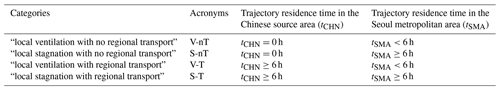Despite the various potential factors like emissions, atmospheric chemistry, and meteorology that can affect the gas and particulate air quality, the simple categorization according to the residence time of backward trajectories could reveal different characteristics in chemical species and meteorological factors associated with the local stagnation and the regional transport of air pollutants. For example, the average concentrations of primary gaseous pollutants (SO2, NO2, and CO) of the “local stagnation with regional transport” (S-T) group are significantly higher than those of the “local stagnation with no regional transport” (S-nT) group (p≤0.001), although average local meteorological factors between the two groups do not show significant differences (Table 2 and Figs. 2 and 3). On the other hand, the lower wind speed and shallower BLH were seen in the local stagnation (S-T and S-nT) groups compared with the local-ventilation (V-T and V-nT) groups (Fig. 2), and the “local stagnation with regional transport” (S-T) group shows significantly higher levels of SO2, NO2, and CO in comparison with the “local ventilation with regional transport” (V-T) group, probably related to accumulation in the stagnant condition. Note that although the present study used a part of total daily data for which OM concentrations are available (n=118), composite averages and differences in meteorological variables and air pollutant concentrations among the four categorized groups using the total daily data including the OM-unavailable dates (n=210) showed the same characteristics as shown in Figs. 2 and 3 (Fig. S5).

Table 2The average and standard deviation of PM2.5 chemical composition, meteorological factors, related gas concentrations, thermodynamic model results and gas-particle partitioning ratios, and mass concentration ratios to wet PM2.5 (PM2.5  wet) for the “local ventilation with no regional transport” (V-nT) group, the “local stagnation with no regional transport” (S-nT) group, the “local ventilation with regional transport” (V-T) group, and the “local stagnation with regional transport” (S-T) group and p values derived from Welch's t test for the composite differences between the S-T and S-nT groups and between the S-T and V-T groups.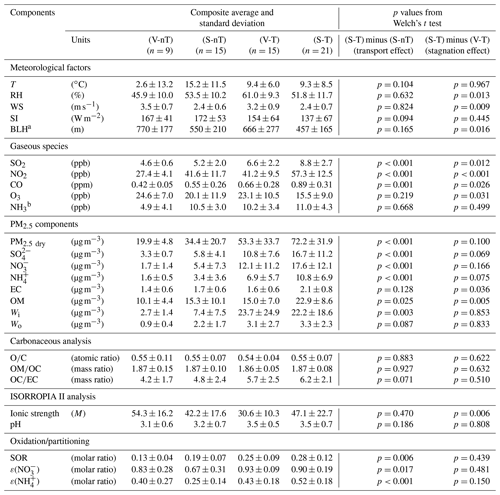a Reanalysis data from the ERA-Interim at 37.5 N, 127.0 E. b Statistically reconstructed data based on Phan et al. (2013). T: temperature; RH: relative humidity;
WS: wind speed; SI: solar irradiance; BLH: boundary layer height; EC: elemental carbon; OM: organic matter; Wi: aerosol liquid water (ALW) content associated with inorganic species; Wo: ALW content associated with OM; OC: organic carbon; SOR: sulfur oxidation ratio; ε(${\mathrm{NO}}_{\mathrm{3}}^{-}$): nitrate partitioning ratio; ε(${\mathrm{NH}}_{\mathrm{4}}^{+}$): ammonium partitioning ratio.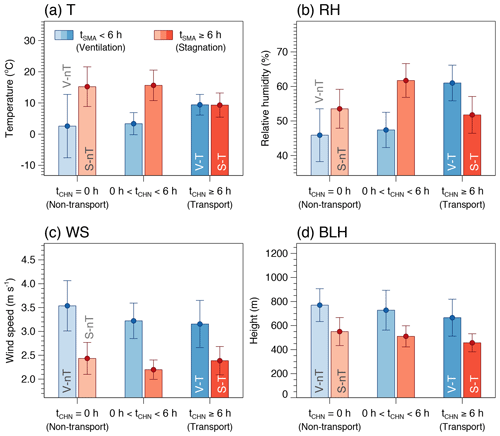Figure 2Averages and confidence intervals at 95 % of (a) temperature (T), (b) relative humidity (RH), (c) wind speed (WS), and (d) boundary layer height (BLH) for the six case groups categorized by the ranges of the average daily residence time of backward trajectories in the Seoul metropolitan area (tSMA) and in the North China Plain and Yangtze River Delta (tCHN).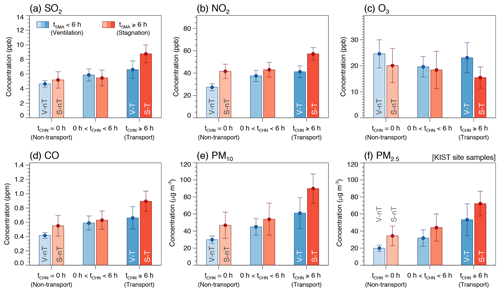Figure 3Averages and confidence intervals at 95 % of daily average concentrations of (a) SO2, (b) NO2, (c) O3, (d) CO, and (e) PM10, together with (f) PM2.5 measured at the KIST site in this study (dry PM2.5) for the six case groups categorized by ranges of the average daily residence time of backward trajectories in the Seoul metropolitan area (tSMA) and in the North China Plain and Yangtze River Delta (tCHN).

## 2.3 Prediction of ALW content and pH

The ISORROPIA II thermodynamic model was run in forward mode to estimate ALW content associated with inorganic species, pH, and the equilibrium gas–particle partitioning based on the daily PM2.5 ionic compositions, RH, and temperature. ISORROPIA II uses total (gas- plus aerosol-phase) measurements as inputs, under the metastable assumption (no solid precipitates). Recent validation studies demonstrate that the forward mode is influenced less by measurement errors and gives a more accurate pH than the reverse mode, which uses only the aerosol-phase composition as an input (Hennigan et al., 2015; Song et al., 2018).

The forward mode of ISORROPIA II requires the total NH3 (gas-phase NH3 plus particulate ${\mathrm{NH}}_{\mathrm{4}}^{+}$), total HNO3 (HNO3 plus particulate ${\mathrm{NO}}_{\mathrm{3}}^{-}$), and total Cl (gas-phase HCl plus particulate Cl) concentrations, as well as the particulate ${\mathrm{SO}}_{\mathrm{4}}^{\mathrm{2}-}$, K+, Ca2+, Na+, and Mg2+ concentrations. However, the ambient NH3, HNO3, and HCl data were not available for this study. To overcome this problem with the input data, we used statistically reconstructed NH3 data and then estimated HNO3 using a thermodynamic model.

Firstly, the daily NH3 concentrations in Seoul from January 2012 to December 2014 were reconstructed using the statistical characteristics of a year-long record of NH3 at the Gwangjin site (37.545 N, 127.096 E) in Seoul for the preceding year (346 d between September 2010 and August 2011; Phan et al., 2013). There were small interannual changes in the estimated NH3 emission in Seoul (Fig. S6; NIER, 2018), and the two sites both share similar environments located downwind from the downtown core under the prevailing westerlies and surrounded by residential and small urban green areas (Fig. S1). Therefore, we assumed that the statistical characteristics of both sites and periods would be similar despite the temporal gap (∼1–3 years) and spatial distance (∼7.8 km) between this study and Phan et al. (2013). We built a multiple linear regression model of NH3 that retains statistical characteristics of the measured NH3 such as the annual average and standard deviation of 10.9±4.25 ppb and the significant linear correlations (p<0.05) with temperature, RH, wind speed, and SO2, NO2, and CO concentrations (Text S1 and Table S1 in the Supplement). The reconstructed NH3 concentration is seasonally high during the warm season as reported by Phan et al. (2013) and shows high peaks on the polluted days with stagnant condition in the cold season (Fig. S7).

In the next step, the ISORROPIA II calculation was performed, using the reconstructed NH3 and measured ${\mathrm{NH}}_{\mathrm{4}}^{+}$ as the total equivalent NH3, and the measured ${\mathrm{NO}}_{\mathrm{3}}^{-}$ alone as the total HNO3, to estimate the HNO3${\mathrm{NO}}_{\mathrm{3}}^{-}$ partitioning ratio. Then, using both the measured ${\mathrm{NO}}_{\mathrm{3}}^{-}$ and the ratio between the HNO3 and ${\mathrm{NO}}_{\mathrm{3}}^{-}$ predicted from the initial result, we estimated the ambient HNO3. Note that we regarded the ${\mathrm{HNO}}_{\mathrm{3}}/{\mathrm{NO}}_{\mathrm{3}}^{-}$ ratio as approximately independent of the total HNO3. Although the nitrate partitioning depends on temperature, ALW content, and pH in general, the ${\mathrm{HNO}}_{\mathrm{3}}/{\mathrm{NO}}_{\mathrm{3}}^{-}$ ratio is affected only by pH because both temperature and ALW are independent variables in this step. In the existence of excess NH3 as we assumed for Seoul (∼10 ppb at the first step), changes in pH by total HNO3 are limited owing to the buffering effect of NH3${\mathrm{NH}}_{\mathrm{4}}^{+}$ partitioning (Weber et al., 2016; Guo et al., 2018; Lim et al., 2020). The pH, nitrate partitioning ratio [ε(${\mathrm{NO}}_{\mathrm{3}}^{-}$) =${\mathrm{NO}}_{\mathrm{3}}^{-}/\left({\mathrm{HNO}}_{\mathrm{3}}+{\mathrm{NO}}_{\mathrm{3}}^{-}\right)$], and the ammonium partitioning ratios [ε(${\mathrm{NH}}_{\mathrm{4}}^{+}$) =${\mathrm{NH}}_{\mathrm{4}}^{+}/\left({\mathrm{NH}}_{\mathrm{3}}+{\mathrm{NH}}_{\mathrm{4}}^{+}\right)$] from the ISORROPIA simulations with and without gas-phase HNO3 information show negligible differences to each other (Fig. 4d–f), unlike the significant role of additional NH3 information in increasing pH and ε(${\mathrm{NO}}_{\mathrm{3}}^{-}$) and decreasing ε(${\mathrm{NH}}_{\mathrm{4}}^{+}$) (Fig. 4a–c).

Finally, we repeated the ISORROPIA simulation with both total NH3 and total HNO3 estimated from the previous steps. Here we did not consider HCl–Cl partitioning because the Cl fraction in dry PM2.5 (∼1 % on average) is significantly smaller than the ${\mathrm{NO}}_{\mathrm{3}}^{-}$ and ${\mathrm{NH}}_{\mathrm{4}}^{+}$ fractions (∼18 % and ∼12 % on average, respectively).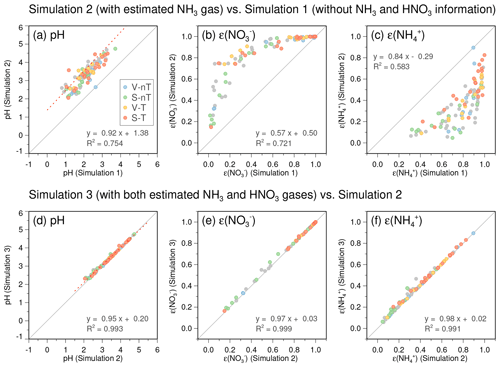Figure 4Comparisons of the predicted pH, nitrate partitioning ratio $\left[\mathit{\epsilon }\left({\mathrm{NO}}_{\mathrm{3}}^{-}\right)={\mathrm{NO}}_{\mathrm{3}}^{-}/\left({\mathrm{HNO}}_{\mathrm{3}}+{\mathrm{NO}}_{\mathrm{3}}^{-}\right)\right]$, and ammonium partitioning ratio $\left[\mathit{\epsilon }\left({\mathrm{NH}}_{\mathrm{4}}^{+}\right)={\mathrm{NH}}_{\mathrm{4}}^{+}/\left({\mathrm{NH}}_{\mathrm{3}}+{\mathrm{NH}}_{\mathrm{4}}^{+}\right)\right]$ between (a–c) the simulation without gas-phase NH3 and HNO3 information (using ${\mathrm{NH}}_{\mathrm{4}}^{+}$ and ${\mathrm{NO}}_{\mathrm{3}}^{-}$ as the total NH3 and total HNO3; Simulation 1) and the simulation with reconstructed NH3 (using NH3 + ${\mathrm{NH}}_{\mathrm{4}}^{+}$ as the total NH3 but only ${\mathrm{NO}}_{\mathrm{3}}^{-}$ as the total HNO3; Simulation 2) and between (d–f) Simulation 2 and the simulation with both estimated gas-phase NH3 and HNO3 information (this study; Simulation 3). Filled circles in light blue, light green, light yellow, and light red colors represent daily data and belong to the “local ventilation with no regional transport” (V-nT) group, “local stagnation with no regional transport” (S-nT) group, “local ventilation with regional transport” (V-T) group, and “local stagnation with regional transport” (S-T) group, respectively. Gray solid lines indicate a 1-to-1 relationship.

Although there are uncertainties in the reconstructed NH3 and HNO3 due to lack of direct measurements, their impact on the estimation of inorganic ALW and particle pH is small enough to utilize them for the thermodynamic analysis in this study. The good agreement between the predicted and statistically reconstructed NH3 concentrations (R2∼0.95), as well as between the predicted and measured ${\mathrm{SO}}_{\mathrm{4}}^{\mathrm{2}-}$, ${\mathrm{NO}}_{\mathrm{3}}^{-}$, and ${\mathrm{NH}}_{\mathrm{4}}^{+}$ concentrations (R2>0.95; Fig. S8), ensures small errors in water contents of individual inorganic species. Since water uptake by inorganic aerosol in ISORROPIA II is based on the Zdanovskii–Stokes–Robinson (ZSR) mixing rule (Stokes and Robinson, 1966), which approximates the water content of a mixture as a sum of water contents of individual salts at the same RH (Fountoukis and Nenes, 2007), the expected error in Wi induced by potential errors in NH3 and HNO3 would also be small. Regarding pH, the buffering effect of semi-volatile NH3 partitioning reduces the sensitivity of pH to excess NH3 in the NH3-rich conditions like Seoul (Weber et al., 2016; Guo et al., 2018; Lim et al., 2020). We conducted ISORROPIA simulations with various NH3 levels (0–50 ppb) applied to all the measurement days as fixed values to further explore the sensitivity of pH, ε(${\mathrm{NO}}_{\mathrm{3}}^{-}$), and ε(${\mathrm{NH}}_{\mathrm{4}}^{+}$) to NH3. Good correlations between results from the daily varied-NH3 simulation and the fixed-NH3 simulations show that potential errors induced by the estimation of daily NH3 levels will be small if NH3 concentrations are nonzero (Fig. 5a, c, and e). The slopes of pH, ε(${\mathrm{NO}}_{\mathrm{3}}^{-}$), and ε(${\mathrm{NH}}_{\mathrm{4}}^{+}$) curves to the increase in fixed-NH3 level become gradually flat at over 5 ppb (Fig. 5b, d, and f), and this indicates that the potential errors in reconstructed NH3 will not significantly change the results in this study.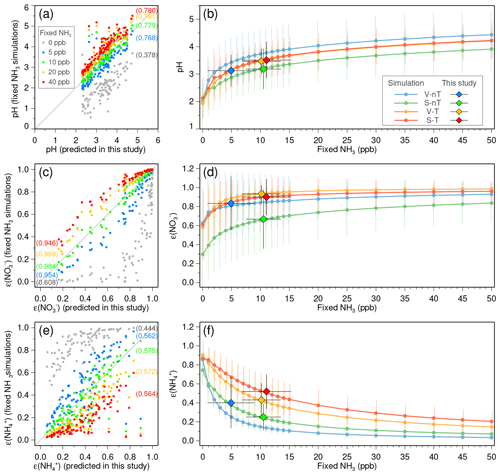Figure 5Sensitivity of (a–b) predicted pH, (c–d) nitrate partitioning ratio $\left[\mathit{\epsilon }\left({\mathrm{NO}}_{\mathrm{3}}^{-}\right)={\mathrm{NO}}_{\mathrm{3}}^{-}/\left({\mathrm{HNO}}_{\mathrm{3}}+{\mathrm{NO}}_{\mathrm{3}}^{-}\right)\right]$, and (e–f) ammonium partitioning ratio $\left[\mathit{\epsilon }\left({\mathrm{NH}}_{\mathrm{4}}^{+}\right)={\mathrm{NH}}_{\mathrm{4}}^{+}/\left({\mathrm{NH}}_{\mathrm{3}}+{\mathrm{NH}}_{\mathrm{4}}^{+}\right)\right]$ to gas-phase ammonia (NH3) concentrations. (a, c, e) Comparisons between the simulation in this study (using the daily reconstructed NH3 concentrations) and the simulations with the various fixed-NH3 levels (colored dots in gray, blue, green, yellow, and red for 0, 5, 10, 20, and 40 ppb, respectively). Values in round brackets are the R-squared values of the linear regressions. Gray solid lines indicate a 1-to-1 relationship. (b, d, f) Average (solid circles) and standard deviation (vertical bars) of pH, $\mathit{\epsilon }\left({\mathrm{NO}}_{\mathrm{3}}^{-}\right)$, and $\mathit{\epsilon }\left({\mathrm{NH}}_{\mathrm{4}}^{+}\right)$ for the “local ventilation with no regional transport” (V-nT) group (light blue), “local stagnation with no regional transport” (S-nT) group (light green), “local ventilation with regional transport” (V-T) group (light yellow), and “local stagnation with regional transport” (S-T) group (light red) with respect to the various fixed-NH3 levels from 0 to 50 ppb. Averages and standard deviations of the reconstructed NH3 concentrations and obtained pH, $\mathit{\epsilon }\left({\mathrm{NO}}_{\mathrm{3}}^{-}\right)$, and $\mathit{\epsilon }\left({\mathrm{NH}}_{\mathrm{4}}^{+}\right)$ for the V-nT, S-nT, V-T, and S-T groups are represented as blue, green, yellow, and red diamonds with horizontal and vertical bars.

Since ALW content associated with OM (Wo) is not considered in ISORROPIA II, we estimated it according to κ-Köhler theory and the ZSR mixing rule (Nguyen et al., 2015, and references therein) using the following equation:

$\begin{array}{}\text{(1)}& {W}_{\mathrm{o}}={V}_{\mathrm{o}}{\mathit{\kappa }}_{\mathrm{org}}\frac{{a}_{\mathrm{w}}}{\mathrm{1}-{a}_{\mathrm{w}}},\end{array}$

where Vo is the volume of OM (µm3 cm−3), which is obtained from OM concentration (µg m−3) divided by a typical organic density of 1.4 g cm−3 (Turpin and Lim, 2001), κorg is the hygroscopicity parameter (dimensionless) calculated from the parameterized relationship of ${\mathit{\kappa }}_{\mathrm{org}}=\left(\mathrm{0.29}±\mathrm{0.05}\right)\cdot \left(\mathrm{O}/\mathrm{C}\right)$ for the range of O∕C from 0.3 to 0.6 (Chang et al., 2010), and aw is water activity (dimensionless) that is assumed to be equivalent to RH (Nguyen et al., 2016).

3 Results and discussion

## 3.1 Effects of regional transport and local stagnation on PM2.5 composition

Average concentrations of PM2.5 and chemical components were highest in the “local stagnation with regional transport” (S-T) group (e.g., PM2.5  dry of 72.2 µg m−3) and lowest in the “local ventilation with no regional transport” (V-nT) group (e.g., PM2.5  dry of 19.9 µg m−3), and the average concentrations for the “local ventilation with regional transport” (V-T) group and the “local stagnation with no regional transport” (S-nT) groups (e.g., PM2.5  dry of 53.3 and 34.4 µg m−3, respectively) were located between those of the S-T and V-nT groups (Table 2 and Fig. 3f). The composite difference between the regional-transport (V-T and S-T) groups and no regional-transport (V-nT and S-nT) groups reveals the regional effect of transported haze particles and precursors from the North China Plain and Yangtze River Delta, while that between the local-stagnation (S-T and S-nT) groups and local-ventilation (V-T and V-nT) groups shows the effect of accumulation or diffusion of both local and transported pollutants in the Seoul metropolitan area. Thus, the significant difference in each PM2.5 component between the S-T and V-nT groups (Fig. 6) indicates both contributions of local stagnant conditions over the Seoul metropolitan area and regional transport from the Chinese source area to the exacerbation of haze pollution in Seoul.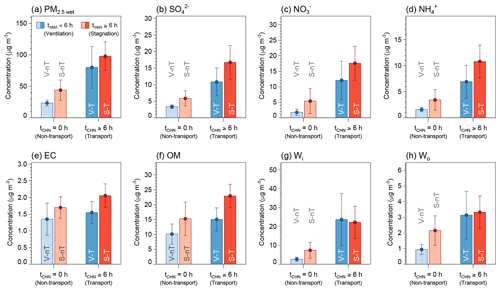Figure 6Average concentrations and confidence intervals at 95 % of (a) wet PM2.5 (PM2.5  wet), (b) sulfate (${\mathrm{SO}}_{\mathrm{4}}^{\mathrm{2}-}$), (c) nitrate (${\mathrm{NO}}_{\mathrm{3}}^{-}$), (d) ammonium (${\mathrm{NH}}_{\mathrm{4}}^{+}$), (e) elemental carbon (EC), (f) organic matter (OM), (g) inorganic ALW (Wi), and (h) organic ALW (Wo) for the “local ventilation with no regional transport” (V-nT) group (light blue), “local stagnation with no regional transport” (S-nT) group (light red), “local ventilation with regional transport” (V-T) group (darker light blue), and “local stagnation with regional transport” (S-T) group (darker light red) categorized by ranges of the average daily residence time of backward trajectories in the Seoul metropolitan area (tSMA) and in the North China Plain and Yangtze River Delta (tCHN).

The average concentrations of gaseous precursors like SO2 and NO2 for the S-T group were about 2 times higher than those for the V-nT group (Fig. 3), while ${\mathrm{SO}}_{\mathrm{4}}^{\mathrm{2}-}$, ${\mathrm{NO}}_{\mathrm{3}}^{-}$, ${\mathrm{NH}}_{\mathrm{4}}^{+}$, and inorganic ALW increased by 5–10 times (Fig. 6). In contrast, average OM concentration for the S-T group was 2 times higher than that for the V-nT group, similarly to CO, and organic ALW of the S-T group showed a relatively smaller increase (∼4 times of the V-nT group) than inorganic ALW (∼8 times of the V-nT group). Therefore, the PM2.5 increase in Seoul seems to be induced not only by the physical and dynamical processes like transport or accumulation of air pollutants but also by the chemical process like secondary aerosol formation, particularly related to the increase in inorganic species and ALW.

A statistically significant difference (p<0.05) between the S-T and S-nT groups (effect of regional transport in the local stagnant condition) was observed in SIA species (${\mathrm{SO}}_{\mathrm{4}}^{\mathrm{2}-}$, ${\mathrm{NO}}_{\mathrm{3}}^{-}$, and ${\mathrm{NH}}_{\mathrm{4}}^{+}$), OM, and ALW (both Wi and Wo), and that between the S-T and V-T groups (effect of local stagnation in the regional-transport condition) was seen for EC and OM (Table 2). Thus, although both regional transport and local stagnation can induce high concentrations of PM2.5 and its chemical components of the S-T group, the effect of regional transport is more significant for the increase in inorganic species and water content, while the effect of local stagnation is more significant for the increase in carbonaceous species. The sulfur oxidation ratio [SOR $={\mathrm{SO}}_{\mathrm{4}}^{\mathrm{2}-}/\left({\mathrm{SO}}_{\mathrm{2}}+{\mathrm{SO}}_{\mathrm{4}}^{\mathrm{2}-}\right)$], nitrate partitioning ratio [ε(${\mathrm{NO}}_{\mathrm{3}}^{-}$)], and ammonium partitioning ratio [ε(${\mathrm{NH}}_{\mathrm{4}}^{+}$)] of the S-T group, which were significantly higher than that of the S-nT group (p<0.05) but did not clearly differ from that of the V-T group, indicate that the increase in SIA through the regional-transport effect is closely associated with the enhanced oxidation (e.g., from NOx to HNO3) or partitioning of inorganic species into the particle phase.

Note that OM concentration seems to be increased by both effects of regional transport and local stagnation (Table 2 and Fig. 6f). However, the average estimates of the O∕C ratio (∼0.55) and OM∕OC ratio (∼1.87), which are in between semi-volatile oxygenated OA (SV-OOA) and low-volatility oxygenated OA (LV-OOA), are similar for the four categorized groups (Table 2). Together with weak correlations of the O∕C ratio with wind speed ($r=-\mathrm{0.093}$) and BLH ($r=-\mathrm{0.172}$) in total daily data (n=118), this suggests that aging and oxidation of SOA in Seoul was not much dependent on external conditions like stagnation or transport. Rather, significant correlations of the O∕C ratio with temperature ($r=-\mathrm{0.531}$) and solar irradiance ($r=-\mathrm{0.370}$) indicate the winter-high/summer-low seasonality of the secondary fraction in OA. Such temperature-dependent SOA fraction can be also found in OC∕EC ratio ($r=-\mathrm{0.638}$ for temperature and $r=-$0.469 for solar irradiance), which can be regarded as an index for secondary OC, and is consistent with partitioning theory (Takekawa et al., 2003).

## 3.2 Fractional changes of ALW and SIA by effects of regional transport and local stagnation

The chemical mass fraction of PM2.5 is expected to be affected by source characteristics (emissions) and chemical process (secondary aerosol formation), but not by the physical and dynamical processes (transport and accumulation), which affect the mass concentration of PM2.5. Thus, the mass fraction of each component is helpful to understand the source and chemical characteristics of each categorized group.

In terms of the component fraction, PM2.5 of the “local stagnation with regional transport” (S-T) group can be characterized by inorganic-rich, wet particles, while that of the “local ventilation with no regional transport” (V-nT) group was relatively organic-richer and drier (Fig. 7). Estimated wet PM2.5 (including ALW of both Wi and Wo; PM2.5  wet) of the S-T group contained larger fractions of SIA species (${\mathrm{SO}}_{\mathrm{4}}^{\mathrm{2}-}$, ${\mathrm{NO}}_{\mathrm{3}}^{-}$, and ${\mathrm{NH}}_{\mathrm{4}}^{+}$) and ALW but a smaller OM fraction (46 % SIA, 23 % OM, and 26 % ALW) than that of the V-nT group (29 % SIA, 43 % OM, and 15 % ALW). Comparisons with the “local ventilation with regional transport” (V-T) group (37 % SIA, 19 % OM, and 33 % ALW) and the “local stagnation with no regional transport” (S-nT) group (34 % SIA, 35 % OM, and 21 % ALW) show that the effect of regional transport increases inorganic and water fractions, while the effect of local stagnation raises organic fraction of the total PM2.5  wet. Note that the increase in ALW fraction from the V-nT (15 %) to V-T (33 %) groups is much larger than that from the S-nT (21 %) to S-T (26 %) groups because of the significantly higher increase in RH from the V-nT (46 %) to V-T (61 %) groups compared to that from the S-nT (54 %) to S-T (52 %) groups. The relationship between RH and ALW, as well as hygroscopic properties of Seoul haze particles, will be further discussed in the next section.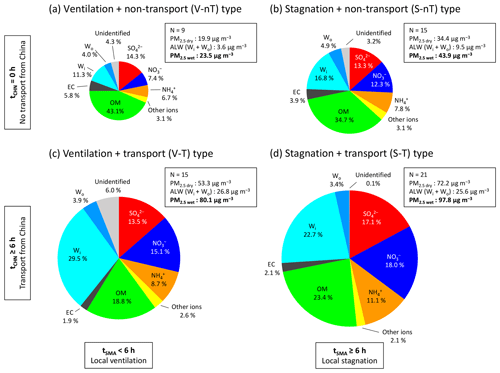Figure 7Average chemical compositions of wet PM2.5 (PM2.5  wet) for the (a) “local ventilation with no regional transport” (V-nT) group, (b) “local stagnation with no regional transport” (S-nT) group, (c) “local ventilation with regional transport” (V-T) group, and (d) “local stagnation with regional transport” (S-T) group. Fractional species are sulfate (red), nitrate (dark blue), ammonium (orange), organic matter (green), elemental carbon (black), inorganic ALW (light blue), organic ALW (darker light blue), and other ions (chloride, sodium, potassium, magnesium, and calcium; yellow). The size of each circle is proportional to the PM2.5  wet concentration.

The ${\mathrm{SO}}_{\mathrm{4}}^{\mathrm{2}-}$ fraction of dry PM2.5 (excluding Wi and Wo; PM2.5  dry) was ∼17 % for the no regional-transport (V-nT and S-nT) groups and about 20 % and 23 % for the V-T and S-T groups, respectively. The ${\mathrm{SO}}_{\mathrm{4}}^{\mathrm{2}-}$ fraction in the no regional-transport groups is close to the background fraction of ${\mathrm{SO}}_{\mathrm{4}}^{\mathrm{2}-}$ in PM2.5  dry in Seoul, and this fraction is comparable to the “local sulfate source” contribution of 21.7 % (mostly in the form of ammonium sulfate and equivalent to the ${\mathrm{SO}}_{\mathrm{4}}^{\mathrm{2}-}$ contribution of ∼16 %) identified by the previous source apportionment study on PM2.5 in Seoul (Kim et al., 2016). A previous field study conducted on the multiday haze episode showed that the ${\mathrm{SO}}_{\mathrm{4}}^{\mathrm{2}-}$ concentration at the upwind background site over the Yellow Sea (Deokjeok Island; 37.233 N, 126.149 E) was nearly the same as Seoul ${\mathrm{SO}}_{\mathrm{4}}^{\mathrm{2}-}$ at the regional-transport stage of haze or the clean period and even kept ∼50 % of the Seoul ${\mathrm{SO}}_{\mathrm{4}}^{\mathrm{2}-}$ concentration at the local-stagnation stage of the haze episode (Seo et al., 2017). Thus, together with considering the small SO2 emission in the Seoul metropolitan area compared to the North China Plain (Li et al., 2017; NIER, 2018) and the SO2 increase through the regional-transport effect (regional-transport groups minus no regional-transport groups; ∼2–4 ppb), the majority of ${\mathrm{SO}}_{\mathrm{4}}^{\mathrm{2}-}$ in the regional-transport (V-T and S-T) groups seems to be directly transported from China or produced during the transport from China before arriving at the Seoul metropolitan area.

The ${\mathrm{NO}}_{\mathrm{3}}^{-}$ fraction of PM2.5  dry was only ∼9 % for the V-nT group but increased to 16 % and 23 % for the S-nT and V-T groups and reached more than 24 % for the S-T group. Interestingly, the fractional increase through the local-stagnation effect (local-stagnation groups minus local-ventilation groups; ∼2 %–7 %) is much smaller than that through the regional-transport effect (regional-transport groups minus no regional-transport groups; ∼9 %–14 %) despite the same increase in NO2 concentration (∼15 ppb; Table 2) through two different effects. Seoul is one of the largest sources of NOx in Korea, but the previous study using satellite NOx column data with the Lagrangian model demonstrated that the Chinese contribution to the NO2 columns over Korea reaches up to ∼50 %–70 % (Lee et al., 2014). However, the impact of regional transport on the increase in ${\mathrm{NO}}_{\mathrm{3}}^{-}$ fraction, which is ∼2 times higher than that of the local stagnation, indicates a nonlinear effect of regional transport on the ${\mathrm{NO}}_{\mathrm{3}}^{-}$ increase in Seoul haze.

The ${\mathrm{NH}}_{\mathrm{4}}^{+}$ fraction of PM2.5  dry was 8 % for the V-nT group, 10 % for the S-nT group, 13 % for the V-T group, and 15 % for the S-T group. The higher fractional increase in ${\mathrm{NH}}_{\mathrm{4}}^{+}$ through the regional-transport effect (T-minus nT-groups; 5 %) compared to that through the local-stagnation effect (S-minus V-groups; 2 %) results from the higher increase in both ${\mathrm{SO}}_{\mathrm{4}}^{\mathrm{2}-}$ and ${\mathrm{NO}}_{\mathrm{3}}^{-}$ aerosols related to the regional-transport effect.

## 3.3 Synergistic effect of transported hygroscopic particles on SIA in the cold season

The high-concentration, inorganic-rich, and wet particles (high SIA and ALW fractions of PM2.5  wet) mostly belong to the regional-transport (V-T and S-T) groups, of which backward trajectories originated from the upwind regional source area (the North China Plain and Yangtze River Delta), while relatively low-concentration, organic-rich dry particles (high OM fraction of PM2.5  wet) belong to the “local ventilation with no regional transport” (V-nT) group (Fig. 8a and b). The ALW fraction is a function of RH and also increases with the SIA fraction at the same RH (Fig. 8c), similar to the Beijing haze (Wu et al., 2018). This indicates that Seoul haze is hygroscopic and the major driver of its hygroscopicity is inorganic species. Since the SIA fraction was relatively higher in the regional-transport groups compared to that in the no regional transport groups, the hygroscopic properties of PM2.5 in Seoul were more clearly revealed in the regional-transport groups (Fig. 8d). Therefore, the ALW fraction in the transported particles (e.g., the regional-transport groups) was higher than that in the particles of local origin (e.g., the “local stagnation with no regional transport” (S-nT) group).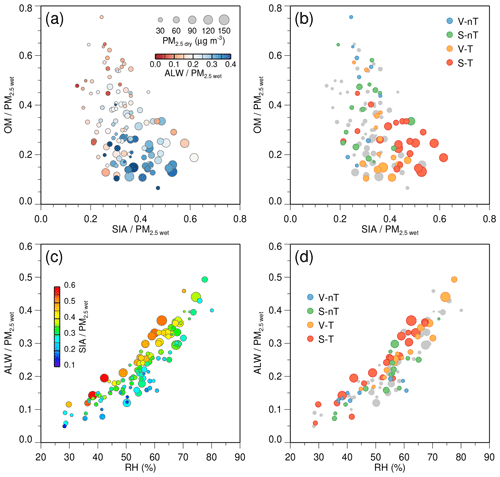Figure 8(a–b) Scatterplots of the secondary inorganic aerosol (SIA) fraction to wet PM2.5 (SIA∕PM2.5  wet) ratio versus the organic matter (OM) fraction to wet PM2.5 (OM∕PM2.5  wet) ratio, colored according to (a) the aerosol liquid water (ALW) to wet PM2.5 (ALW∕PM2.5  wet) ratio and (b) the categories for each case. (c–d) Scatterplots of ALW∕PM2.5  wet versus relative humidity (RH), colored according to (c) SIA∕PM2.5  wet ratio and (d) the categories. The size of each circle is proportional to the dry PM2.5 (PM2.5  dry) concentration.

The concentrations of ${\mathrm{SO}}_{\mathrm{4}}^{\mathrm{2}-}$, ${\mathrm{NO}}_{\mathrm{3}}^{-}$, and ${\mathrm{NH}}_{\mathrm{4}}^{+}$ increase with their gas-phase precursors (SO2, NO2, and NH3) in Seoul, except for the V-nT group. However, the regression slopes of the ${\mathrm{SO}}_{\mathrm{4}}^{\mathrm{2}-}$, ${\mathrm{NO}}_{\mathrm{3}}^{-}$, and ${\mathrm{NH}}_{\mathrm{4}}^{+}$ with respect to their precursors in the regional-transport groups are steeper than the slope of the S-nT group (Fig. 9). Such a higher sensitivity of the increase in ${\mathrm{SO}}_{\mathrm{4}}^{\mathrm{2}-}$, ${\mathrm{NO}}_{\mathrm{3}}^{-}$, and ${\mathrm{NH}}_{\mathrm{4}}^{+}$ to the gas-phase precursors of the regional-transport groups is related to the higher ratios of sulfur oxidation (SOR) and partitioning [ε(${\mathrm{NO}}_{\mathrm{3}}^{-}$) and ε(${\mathrm{NH}}_{\mathrm{4}}^{+}$)] compared to the S-nT group (Fig. 10b, f, and j). Since the average concentrations of total sulfur ($={\mathrm{SO}}_{\mathrm{2}}+{\mathrm{SO}}_{\mathrm{4}}^{\mathrm{2}-}$), total HNO3, and total NH3 are highest in the “local stagnation with regional transport” (S-T) group due to a combination of remote precursors transported from China and local precursors accumulated in the stagnant condition (Fig. 10a, e, and i), the high SOR, ε(${\mathrm{NO}}_{\mathrm{3}}^{-}$), and ε(${\mathrm{NH}}_{\mathrm{4}}^{+}$) of the regional-transport groups induced the highest average ${\mathrm{SO}}_{\mathrm{4}}^{\mathrm{2}-}$, ${\mathrm{NO}}_{\mathrm{3}}^{-}$, and ${\mathrm{NH}}_{\mathrm{4}}^{+}$ concentrations of the S-T group (Table 2).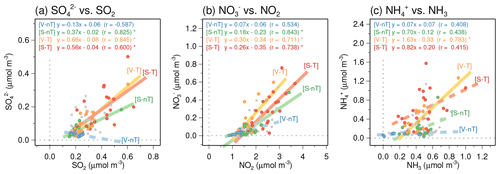Figure 9Scatterplots of (a) ${\mathrm{SO}}_{\mathrm{4}}^{\mathrm{2}-}$ versus SO2, (b) ${\mathrm{NO}}_{\mathrm{3}}^{-}$ versus NO2, and (c) ${\mathrm{NH}}_{\mathrm{4}}^{+}$ versus the reconstructed ammonia (NH3). Filled circles in blue, green, yellow, and red colors represent daily data belonging to the “local ventilation with no regional transport” (V-nT) group, “local stagnation with no regional transport” (S-nT) group, “local ventilation with regional transport” (V-T) group, and “local stagnation with regional transport” (S-T) group, respectively. The statistically significant linear regression slopes (p<0.01) for each group are represented by solid lines.

The SOR, ε(${\mathrm{NO}}_{\mathrm{3}}^{-}$), and ε(${\mathrm{NH}}_{\mathrm{4}}^{+}$) averaged for four range classes of temperature and inorganic ALW (Wi) show that all the ratios increased with Wi (Fig. 10d, h, and l), and ε(${\mathrm{NO}}_{\mathrm{3}}^{-}$) and ε(${\mathrm{NH}}_{\mathrm{4}}^{+}$) decreased with temperature (Fig. 10g and k). Changes in Wi from the S-nT group (∼7µg m−3 on average; Wi1) to the regional-transport groups (>20µg m−3 on average; Wi3) increase SOR, ε(${\mathrm{NO}}_{\mathrm{3}}^{-}$), and ε(${\mathrm{NH}}_{\mathrm{4}}^{+}$) by approximately up to 20 % for each ratio. This implies that the transported haze particles in the regional-transport groups, which are richer in inorganics and more hygroscopic than the local haze particles of the S-nT group (Fig. 8), promote SIA production. The aqueous-phase oxidation of SO2 via H2O2, O3, NO2, and transition metal ion (TMI) pathways (Harris et al., 2013; Cheng et al., 2016; Wang et al., 2016) and the partitioning of HNO3 and NH3 via dissolution and dissociation in the wet particles (Seinfeld and Pandis, 2016) are effective ways to produce ${\mathrm{SO}}_{\mathrm{4}}^{\mathrm{2}-}$, ${\mathrm{NO}}_{\mathrm{3}}^{-}$, and ${\mathrm{NH}}_{\mathrm{4}}^{+}$. Therefore, ALW in the hygroscopic particles can synergistically increase the inorganic species in a precursor-rich urban area like Seoul.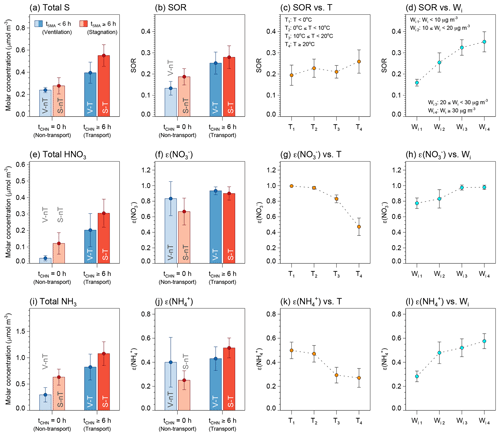Figure 10Average concentrations and confidence intervals at 95 % of (a) total sulfur (= SO2+ ${\mathrm{SO}}_{\mathrm{4}}^{\mathrm{2}-}$), (b–d) sulfur oxidation ratio (SOR), (e) total HNO3 (= HNO3 + ${\mathrm{NO}}_{\mathrm{3}}^{-}$), (f–h) nitrate partitioning ratio [ε(${\mathrm{NO}}_{\mathrm{3}}^{-}$)], (i) total NH3 (= NH3 + ${\mathrm{NH}}_{\mathrm{4}}^{+}$), and (j–l) ammonium partitioning ratio [ε(${\mathrm{NH}}_{\mathrm{4}}^{+}$)]. (a–b, e–f, i–j) Average for the “local ventilation with no regional transport” (V-nT) group (light blue), “local stagnation with no regional transport” (S-nT) group (light red), “local ventilation with regional transport” (V-T) group (darker light blue), and “local stagnation with regional transport” (S-T) group (darker light red). (c, g, k) Average for the temperature (T) ranges of T<0C (T1; n=19), 0 C $\le T<\mathrm{10}$C (T2; n=31), 10 C $\le T<\mathrm{20}$C (T3; n=30), and T≥20C (T4; n=19). (d, h, l) Average for the inorganic ALW (Wi) ranges of Wi<10µg m−3 (Wi1; n=58), 10 µgm−3 $\le {W}_{\mathrm{i}}<\mathrm{20}$µg m−3 (${W}_{\mathrm{i}\mathrm{2}};n=\mathrm{14}$), 20 µgm−3 $\le {W}_{\mathrm{i}}<\mathrm{30}$µg m−3 (Wi3; n=12), and Wi≥30µg m−3 (Wi4; n=15).

In terms of the synergistic increase in ${\mathrm{NO}}_{\mathrm{3}}^{-}$ with ALW, the ratio between ${\mathrm{NO}}_{\mathrm{3}}^{-}$ and ${\mathrm{SO}}_{\mathrm{4}}^{\mathrm{2}-}$ can be an important factor. Hygroscopic uptake of ALW by both ${\mathrm{SO}}_{\mathrm{4}}^{\mathrm{2}-}$ and ${\mathrm{NO}}_{\mathrm{3}}^{-}$ can increase pH through a dilution effect on hydrogen ions (H+). Because ${\mathrm{NO}}_{\mathrm{3}}^{-}$ is a semi-volatile hygroscopic species, the higher pH increased by ALW allows more partitioning of HNO3 gas into the particle phase and takes up more ALW. However, if ${\mathrm{SO}}_{\mathrm{4}}^{\mathrm{2}-}$ is dominant in the particle, such a feedback process will be weakened because ${\mathrm{SO}}_{\mathrm{4}}^{\mathrm{2}-}$ is non-volatile (Guo et al., 2017). The average ${\mathrm{NO}}_{\mathrm{3}}^{-}$-to-${\mathrm{SO}}_{\mathrm{4}}^{\mathrm{2}-}$ molar ratios of the regional-transport groups (1.87 for the S-T group and 1.60 for the V-T group) are higher than that of the no regional-transport groups (1.29 for the S-nT group and 0.81 for the V-nT group). Since ALW, pH, and ε(${\mathrm{NO}}_{\mathrm{3}}^{-}$) in the regional-transport (V-T and S-T) groups are higher than those in the no regional-transport (V-nT and S-nT) groups, this feedback process can explain the synergistic effect of transported particles on the high ${\mathrm{NO}}_{\mathrm{3}}^{-}$ and ALW fractions.

Note that the partitioning of HNO3 and NH3 is also dependent on temperature (T) (Fig. 10g and k). Changes in T from the S-nT group (∼15C on average; T3) to the regional-transport groups (∼9C on average; T2) can also increase ε(${\mathrm{NO}}_{\mathrm{3}}^{-}$) and ε(${\mathrm{NH}}_{\mathrm{4}}^{+}$) by approximately 20 % for each, similar to the effect of inorganic ALW. In Korea, the regional transport of air pollutants from the NCP area is usually accompanied by westerly or northwesterly continental outflow, which induces cold advection, during the cold season (Kim et al., 2018). The lower temperature of the S-T group (∼9C) compared to the S-nT group (∼15C) could lead to the higher sensitivity of the SIA increase to the precursor concentrations in the S-T group (Fig. 9). Interestingly, the increasing SOR through temperature (and also irradiance) is not as significant as that through inorganic ALW (Figs. 10c) despite the high-temperature and strong-irradiance conditions conducive to photochemical oxidation of SO2 in summer. This implies that the observed high ${\mathrm{SO}}_{\mathrm{4}}^{\mathrm{2}-}$ in the S-T group resulted not only from the photochemical gas-phase oxidation but also considerably from the aqueous-phase oxidation of SO2 in the transported wet particles.

## 3.4 Effect of NOx and NH3 control on mitigating haze pollution in Seoul

The ε(${\mathrm{NO}}_{\mathrm{3}}^{-}$) can be analytically calculated according to the solubility and dissociation of gas-phase HNO3 and represented as a sigmoid function (S curve) of pH (Guo et al., 2018; Nah et al., 2018) by the following equation:

$\begin{array}{}\text{(2)}& \begin{array}{rl}& \mathit{\epsilon }\left({\mathrm{NO}}_{\mathrm{3}}^{-}\right)=\\ & \frac{{H}_{{\mathrm{HNO}}_{\mathrm{3}}^{\ast }}{W}_{\mathrm{i}}RT\left(\mathrm{0.987}×{\mathrm{10}}^{-\mathrm{14}}\right)}{{\mathit{\gamma }}_{{\mathrm{H}}^{+}}{\mathit{\gamma }}_{{\mathrm{NO}}_{\mathrm{3}}^{-}}{\mathrm{10}}^{-\mathrm{pH}}+{H}_{{\mathrm{HNO}}_{\mathrm{3}}^{\ast }}{W}_{\mathrm{i}}RT\left(\mathrm{0.987}×{\mathrm{10}}^{-\mathrm{14}}\right)},\end{array}\end{array}$

where $\mathrm{0.987}×{\mathrm{10}}^{-\mathrm{14}}$ is the unit conversion factor from atmospheric pressure and micrograms to SI units, R is the gas constant (8.314 J mol−1 K−1), Wi (µg m−3) is the ALW content associated with inorganic species, and ${H}_{{\mathrm{HNO}}_{\mathrm{3}}^{\ast }}$ is the effective Henry's law constant (products of Henry's law constant for HNO3 gases and the acid dissociation constant for ${\mathrm{HNO}}_{\mathrm{3}}↔{\mathrm{NO}}_{\mathrm{3}}^{-}+{\mathrm{H}}^{+}$) dependent on temperature and pH (mol2 kg−2 atm−1 on a molality basis; Clegg et al., 1998). The product of activity coefficients, ${\mathit{\gamma }}_{{\mathrm{H}}^{+}}{\mathit{\gamma }}_{{\mathrm{NO}}_{\mathrm{3}}^{-}}$, is dependent on both temperature and ionic strength (Kusik and Meissner, 1978; Kim et al., 1993). In this study, daily ${\mathit{\gamma }}_{{\mathrm{H}}^{+}}{\mathit{\gamma }}_{{\mathrm{NO}}_{\mathrm{3}}^{-}}$ was obtained based on daily average ambient temperature and ionic strength from ISORROPIA II and ranged from 0.135 to 0.734, with an average of 0.333±0.150.

The S curve of ε(${\mathrm{NO}}_{\mathrm{3}}^{-}$) as a function of pH is useful for examining the role of inorganic ALW and temperature in HNO3${\mathrm{NO}}_{\mathrm{3}}^{-}$ partitioning and provides insights into the potential HNO3 partitioning by a change in particle pH. In Fig. 11, both a decrease in temperature and an increase in ALW can shift the ε(${\mathrm{NO}}_{\mathrm{3}}^{-}$) curves toward a lower pH, so more HNO3 can exist in the particle phase. Almost 100 % of the total HNO3 exists in the particle phase at the higher pH (>4) in Seoul. Since the gas-phase HNO3 largely formed by the reaction of NO2 with hydroxyl radicals (OH) is highly water-soluble (Seinfeld and Pandis, 2016), the transported wet particles (the regional-transport (V-T and S-T) groups) can easily take up HNO3 gas into the aerosol solution in the NOx-rich (∼60 ppb) environment of Seoul (Vellingiri et al., 2015), particularly during the cold season. Note that ALW can additionally enhance the nitrate radical (NO3)–N2O5 pathway and heterogeneous N2O5 hydrolysis (Bertram et al., 2009). In addition, the high NH3 level (∼10 ppb) in Seoul (Phan et al., 2013) helps further to increase the uptake of HNO3 gas into the aerosol solution (Guo et al., 2018).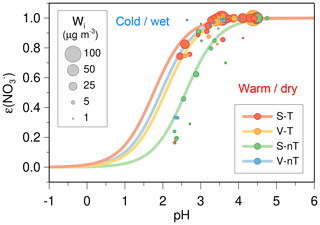Figure 11The calculated sigmoid curves (S curves) and observed ambient gas-particle partitioning ratios (solid circles) for nitrate [ε(${\mathrm{NO}}_{\mathrm{3}}^{-}$)] plotted against the ISORROPIA-predicted particle pH. The blue, green, yellow, and red circles represent the “local ventilation with no regional transport” (V-nT) group, “local stagnation with no regional transport” (S-nT) group, “local ventilation with regional transport” (V-T) group, and “local stagnation with regional transport” (S-T) group, respectively, and the size of each circle is proportional to the concentration of inorganic ALW (Wi). The curves were calculated based on the median values of temperature (T), Wi, and the product of activity coefficient (${\mathit{\gamma }}_{{\mathrm{H}}^{+}}{\mathit{\gamma }}_{{\mathrm{NO}}_{\mathrm{3}}^{-}}$) for each group; T=0.6C, Wi=2.2µg m−3, and ${\mathit{\gamma }}_{{\mathrm{H}}^{+}}{\mathit{\gamma }}_{{\mathrm{NO}}_{\mathrm{3}}^{-}}=\mathrm{0.146}$ for the V-nT group; T=17.5C, Wi=5.6µg m−3, and ${\mathit{\gamma }}_{{\mathrm{H}}^{+}}{\mathit{\gamma }}_{{\mathrm{NO}}_{\mathrm{3}}^{-}}=$ 0.295 for the S-nT group; T= 11.6 C, Wi=16.6µg m−3, and ${\mathit{\gamma }}_{{\mathrm{H}}^{+}}{\mathit{\gamma }}_{{\mathrm{NO}}_{\mathrm{3}}^{-}}=\mathrm{0.458}$ for the V-T group; T=6.9C, Wi=18.0µg m−3, and ${\mathit{\gamma }}_{{\mathrm{H}}^{+}}{\mathit{\gamma }}_{{\mathrm{NO}}_{\mathrm{3}}^{-}}=\mathrm{0.400}$ for the S-T group.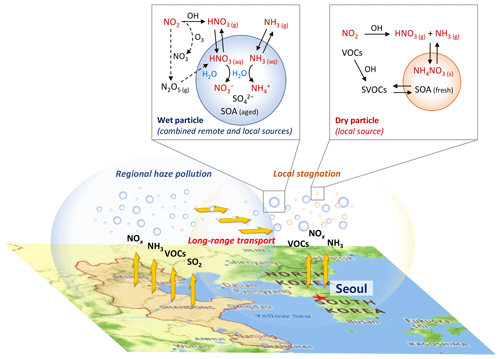Figure 12Schematic of synergistic nitrate partitioning to the particle phase aided by wet particles transported from the remote source area (North China Plain and Yangtze River Delta) in a high NOx and NH3 urban area (Seoul metropolitan area) during the cold season in East Asia. The background map was derived from © Google Maps.

To reduce ammonium nitrate aerosols in Seoul, therefore, two ways can be suggested; the NOx control and the NH3 control. As shown in Fig. 9b, the observed ${\mathrm{NO}}_{\mathrm{3}}^{-}$ concentration is highly correlated with the NO2 level, and this implies that the NOx control will be an effective way to reduce total HNO3 and ${\mathrm{NO}}_{\mathrm{3}}^{-}$ concentrations. Such a direct relationship between NOx control and nitrate aerosol is significant at the condition of ε(${\mathrm{NO}}_{\mathrm{3}}^{-}$) close to 1. Because of the higher ε(${\mathrm{NO}}_{\mathrm{3}}^{-}$) of the transported wet particles, the NOx reduction will more effectively reduce ${\mathrm{NO}}_{\mathrm{3}}^{-}$ in the regional-transport groups compared to the “local stagnation with no regional transport” (S-nT) group. For example, the potential ${\mathrm{NO}}_{\mathrm{3}}^{-}$ reduction by a 10 ppb decrease in ambient NO2 concentration deduced from the linear regression in Fig. 9b can be estimated as being ∼8µg m−3 for the “local ventilation with regional transport” (V-T) group, ∼7µg m−3 for the “local stagnation with regional transport” (S-T) group, and ∼5µg m−3 for the “local stagnation with no regional transport” (S-nT) group. On the other hand, the NH3 control to achieve the low ε(${\mathrm{NO}}_{\mathrm{3}}^{-}$) by lowering the particle pH from the current level (pH of ∼3.5 on average) for the S-T group may cost more than that for the S-nT group because the ε(${\mathrm{NO}}_{\mathrm{3}}^{-}$) in the S-nT group (green circles) starts to decrease rapidly at pH <3.5, while that in the regional-transport groups (yellow circles for the V-T group and red circles for the S-T group) remains relatively high (∼80 %) at the lower pH (∼2.5) (Fig. 11). Since the haze pollution in Seoul usually becomes severe with the regional transport of hygroscopic wet particles (e.g., the S-T group), a greater reduction in the NH3 emissions should be required for the higher-concentration S-T group compared to the lower-concentration S-nT group. However, the benefit of reducing PM2.5 mass concentration by an NH3 control can be canceled out by the adverse effects of strong particle acidity on human health (Fang et al., 2017).

4 Conclusions

Based on PM2.5 chemical speciation, gaseous pollutants, and meteorological data in Seoul together with backward trajectory analysis, the present study investigated chemical compositions and characteristics of urban haze particles in the Asian continental outflow region, according to physical and dynamical conditions such as local stagnant condition in the urban area and regional transport of air pollutants from the remote source area. Although various factors like local emissions of primary pollutants and secondary precursors, atmospheric chemistry related to the secondary formation and aging of aerosols, and meteorological conditions can affect the urban haze pollution, a simple categorization by average residence times of backward trajectories within the local (Seoul metropolitan area) and remote (North China Plain and Yangtze River Delta) source areas showed clearly distinguishable characteristics in concentration and fractional composition of PM2.5 among the local-stagnation/ventilation groups and the regional-transport/non-transport groups. In particular, ALW content associated with inorganic species and particle pH estimated by the ISORROPIA II thermodynamic model helped to show different hygroscopic and inorganic partitioning properties of Seoul haze by the categorized groups.

The measurement group of “local stagnation with regional transport” (S-T) from China is characterized by higher PM2.5 concentration (72±32µg m−3 of PM2.5  dry) and more inorganic-rich and wetter particles (46 % SIA, 23 % OM, and 26 % ALW in PM2.5  wet) in comparison to the measurement group of “local ventilation with no regional transport” (V-nT; 20±5µg m−3 of PM2.5  dry and 29 % SIA, 43 % OM, and 15 % ALW in PM2.5  wet). An increase in SIA from the “local ventilation with no regional transport” (V-nT) group to the “local stagnation with regional transport” (S-T) group (∼7 times from ∼7 to 45 µg m−3) is larger than the OM increase (∼2 times from 10 to 23 µg m−3) but relates closely to the increase in inorganic ALW (∼7 times from ∼3 to 22 µg m−3), indicating inorganic species as a major driver of hygroscopicity. The larger increase in SIA species (${\mathrm{SO}}_{\mathrm{4}}^{\mathrm{2}-}$ from ∼3 to 17 µg m−3 and ${\mathrm{NO}}_{\mathrm{3}}^{-}$ from ∼2 to 18 µg m−3) compared to the increase in gaseous precursors (SO2 from 5 to 9 ppb and NO2 from 27 to 57 ppb) suggests that there is not only the accumulation of local and transported particles but also additional chemical processes for the SIA production in the combination of regional transport and local stagnant conditions.

Further comparisons with the “local stagnation with no regional transport” (S-nT) group and the “local ventilation with regional transport” (V-T) group shows the stronger influence of regional transport rather than local stagnation on the high oxidation and partitioning ratios (SOR, ε(${\mathrm{NO}}_{\mathrm{3}}^{-}$), and ε(${\mathrm{NH}}_{\mathrm{4}}^{+}$)) associated with the SIA increase. SOR, ε(${\mathrm{NO}}_{\mathrm{3}}^{-}$), and ε(${\mathrm{NH}}_{\mathrm{4}}^{+}$) were raised up to ∼20 % by the increase in inorganic ALW in the Seoul haze condition, and this demonstrates an important role of ALW of the transported hygroscopic particles in increasing the SIA fraction. In addition, ε(${\mathrm{NO}}_{\mathrm{3}}^{-}$) and ε(${\mathrm{NH}}_{\mathrm{4}}^{+}$) are decreased through temperature, and thus the transported wet particles can efficiently convert the gas-phase HNO3 and NH3 into the particle-phase ${\mathrm{NO}}_{\mathrm{3}}^{-}$ and ${\mathrm{NH}}_{\mathrm{4}}^{+}$ during the cold season. Therefore, the synergistic effect of transported wet particles and local precursors on the SIA increase and high PM2.5 concentration in Seoul can be most prominent in the cold season, when the continental outflow dominates East Asia and helps transboundary transport of air pollutants to Korea. Since SO2 emissions in Seoul are small, the increased ${\mathrm{SO}}_{\mathrm{4}}^{\mathrm{2}-}$ in the regional-transport condition is likely to be transported from the remote source areas in China or produced during the transport. On the other hand, the high-NOx (∼60 ppb) and NH3-rich (∼10 ppb) conditions in Seoul can promote the uptake of HNO3 into the wet particle.

Most of the severe haze events in Seoul occur in the local stagnant conditions combined with transport of the preceding regional haze (Seo et al., 2017). The transported regional haze particles with a high inorganic fraction and abundant ALW readily take up HNO3 and NH3 gases under the high NOx and NH3 conditions of the urban area and consequently reduce the air quality more than local haze formation without regional transport (Fig. 12). Considering both the high ε(${\mathrm{NO}}_{\mathrm{3}}^{-}$) of the transported wet particles and the low pH required to decrease ε(${\mathrm{NO}}_{\mathrm{3}}^{-}$) in the combined regional-transport–local-stagnation condition, NOx control rather than NH3 control may be a more effective PM2.5 reduction strategy in Seoul.

Our results provide insight into the nonlinear effects of the transported particles and local precursors on urban haze pollution in the regional air quality modeling system. For example, domestic and foreign contributions to PM10 concentration over the Seoul metropolitan area estimated by the brute force method approach show a discrepancy between reductions in domestic and foreign emissions, in particular during the cold season (Kim et al., 2017). The synergistic enhancement of urban haze pollution by the combination of regional and local sources makes a precise estimation of domestic and foreign contributions difficult. This study also shows a need for international cooperation in air quality management.

Code availability

The ISORROPIA II thermodynamic model (Fountoukis and Nenes, 2007) is available on the website of the Laboratory of atmospheric processes and their impacts (LAPI) in the EPFL (https://www.epfl.ch/labs/lapi/isorropia/iso-code-repository/, last access: 25 June 2020). The HYSPLIT model (Stein et al., 2015) is available on the website managed by the NOAA Air Resources Laboratory (https://www.ready.noaa.gov/HYSPLIT.php, last access: 25 June 2020).

Data availability

Daily PM2.5 measurements, chemical analysis, meteorological factors, and ISORROPIA II result data utilized in this study are available at https://drive.google.com/open?id=1hJrwViP_qx23dTuBxLapbWhAn5LrTXMI (last access: 25 June 2020). The hourly data of SO2, NO2, CO, O3, and PM10 concentrations at 34 air quality monitoring sites in Seoul for the analysis period are available on the website managed by the Korea Environment Corporation (2019; https://www.airkorea.or.kr/web/last_amb_hour_data?pMENU_NO=123). The hourly meteorological data of temperature, sea level pressure, relative humidity, wind speed, and solar irradiance at the Seoul weather station for the same period can be found on the website of the KMA (2020; https://data.kma.go.kr/data/grnd/selectAsosRltmList.do?pgmNo=36). The ERA-Interim data (Dee et al., 2011) can be accessed via the European Centre for Medium-Range Weather Forecasts (ECMWF) data server (http://apps.ecmwf.int/datasets/data/interim-full-daily/, last access: 25 June 2020).

Supplement

Author contributions

JS initiated the investigation and performed thermodynamic modeling analyses. JS and YBL extensively discussed the concept. HCJ conducted the field measurement and provided chemical analyses. DY and JYK provided additional feedback on the paper. JS prepared the paper with contributions from all co-authors.

Competing interests

The authors declare that they have no conflict of interest.

Acknowledgements

This research was supported by the Korea Institute of Science and Technology (KIST) and the National Strategic Project – Fine Particle of the National Research Foundation of Korea (NRF) funded by the Ministry of Science and ICT (MSIT), the Ministry of Environment (ME), and the Ministry of Health and Welfare (MOHW) (2017M3D8A1090654). Yong Bin Lim was supported by NRF (2019M3D8A1070941). Daeok Youn was supported by the Basic Science Research Program through the National Research Foundation of Korea (NRF) funded by the Ministry of Education (MOE) (2015R1D1A3A01020130) and Korea Environment Industry & Technology Institute (KEITI) funded by the Korea Ministry of Environment (ME) (2018001310004). The authors are grateful to the editor and two referees for their valuable and constructive comments and suggestions.

Financial support

This research has been supported by the National Strategic Project – Fine Particle of the National Research Foundation of Korea (NRF) funded by the Ministry of Science and ICT (MSIT), the Ministry of Environment (ME), and the Ministry of Health and Welfare (MOHW) (grant no. 2017M3D8A1090654), the National Research Foundation of Korea (NRF) (grant no. 2019M3D8A1070941), the Basic Science Research Program through the National Research Foundation of Korea (NRF) funded by the Ministry of Education (MOE) (grant no. 2015R1D1A3A01020130), and the Korea Environment Industry & Technology Institute (KEITI) funded by the Ministry of Environment (ME) (grant no. 2018001310004).

Review statement

This paper was edited by Barbara Ervens and reviewed by Rodney Weber and one anonymous referee.

References

Asa-Awuku, A., Nenes, A., Gao, S., Flagan, R. C., and Seinfeld, J. H.: Water-soluble SOA from Alkene ozonolysis: composition and droplet activation kinetics inferences from analysis of CCN activity, Atmos. Chem. Phys., 10, 1585–1597, https://doi.org/10.5194/acp-10-1585-2010, 2010.

Ashbaugh, L. L. and Eldred R. A.: Loss of particle nitrate from Teflon sampling filters: Effects on measured gravimetric mass in California and in the IMPROVE network, J. Air Waste Manage., 54, 93–104, https://doi.org/10.1080/10473289.2004.10470878, 2004.

Bertram, T. H., Thornton, J. A., Riedel, T. P., Middlebrook, A. M., Bahreini, R., Bates, T. S., Quinn, P. K., and Coffman, D. J.: Direct observations of N2O5 reactivity on ambient aerosol particles, Geophys. Res. Lett., 36, L19803, https://doi.org/10.1029/2009GL040248, 2009.

Birch, M. and Cary, R: Elemental carbon-based method for monitoring occupational exposures to particulate diesel exhaust, Aerosol Sci. Tech., 25, 221–241, https://doi.org/10.1080/02786829608965393, 1996.

Carlton, A. G. and Turpin, B. J.: Particle partitioning potential of organic compounds is highest in the Eastern US and driven by anthropogenic water, Atmos. Chem. Phys., 13, 10203–10214, https://doi.org/10.5194/acp-13-10203-2013, 2013.

Chang, R. Y.-W., Slowik, J. G., Shantz, N. C., Vlasenko, A., Liggio, J., Sjostedt, S. J., Leaitch, W. R., and Abbatt, J. P. D.: The hygroscopicity parameter (κ) of ambient organic aerosol at a field site subject to biogenic and anthropogenic influences: relationship to degree of aerosol oxidation, Atmos. Chem. Phys., 10, 5047–5064, https://doi.org/10.5194/acp-10-5047-2010, 2010.

Cheng, Y., Zheng, G., Wei, C., Mu, Q., Zheng, B., Wang, Z., Gao, M., Zhang, Q., He, K., Carmichael, G., Pöschl, U., and Su, H: Reactive nitrogen chemistry in aerosol water as a source of sulfate during haze events in China, Sci. Adv., 2, e1601530, https://doi.org/10.1126/sciadv.1601530, 2016.

Chow, J. C., Watson, J. G., Lowenthal, D. H., and Magliano, K. L.: Loss of PM2.5 nitrate from filter samples in central California, J. Air Waste Manage., 55, 1158–1168, https://doi.org/10.1080/10473289.2005.10464704, 2005.

Clegg, S. L., Brimblecombe, P., and Wexler, A. S.: Thermodynamic model of the system H+-${\mathrm{NH}}_{\mathrm{4}}^{+}$- ${\mathrm{SO}}_{\mathrm{4}}^{\mathrm{2}-}$${\mathrm{NO}}_{\mathrm{3}}^{-}$H2O at tropospheric temperatures, J. Phys. Chem. A, 102, 2137–2154, https://doi.org/10.1021/jp973042r, 1998.

Dee, D. P., Uppala, S. M., Simmons, A. J., Berrisford, P., Poli, P., Kobayashi, S., Andrae, U., Balmaseda, M. A., Balsamo, G., Bauer, P., Bechtold, P., Beljaars, A. C. M., van de Berg, L., Bidlot, J., Bormann, N., Delsol, C., Dragani, R., Fuentes, M., Geer, A. J., Haimberger, L., Healy, S. B., Hersbach, H., Hólm, E. V., Isaksen, L., Kållberg, P., Köhler, M., Matricardi, M., McNally, A. P., Monge-Sanz, B. M., Morcrette, J.-J., Park, B.-K., Peubey, C., de Rosnay, P., Tavolato, C., Thépaut, J.-N., and Vitart, F.: The ERA-Interim reanalysis: configuration and performance of the data assimilation system, Q. J. Roy. Meteor. Soc., 137, 553–597, https://doi.org/10.1002/qj.828, 2011.

Fang, T., Guo, H., Zeng, L., Verma, V., Nenes, A., and Weber, R. J.: Highly Acidic Ambient Particles, Soluble Metals, and Oxidative Potential: A Link between Sulfate and Aerosol Toxicity, Environ. Sci. Technol., 51, 2611–2620, https://doi.org/10.1021/acs.est.6b06151, 2017.

Fountoukis, C. and Nenes, A.: ISORROPIA II: a computationally efficient thermodynamic equilibrium model for K+Ca2+Mg2+${\mathrm{NH}}_{\mathrm{4}}^{+}$Na+${\mathrm{SO}}_{\mathrm{4}}^{\mathrm{2}-}$${\mathrm{NO}}_{\mathrm{3}}^{-}$ClH2O aerosols, Atmos. Chem. Phys., 7, 4639–4659, https://doi.org/10.5194/acp-7-4639-2007, 2007.

Guo, H., Liu, J., Froyd, K. D., Roberts, J. M., Veres, P. R., Hayes, P. L., Jimenez, J. L., Nenes, A., and Weber, R. J.: Fine particle pH and gas–particle phase partitioning of inorganic species in Pasadena, California, during the 2010 CalNex campaign, Atmos. Chem., Phys., 17, 5703–5719, https://doi.org/10.5194/acp-17-5703-2017, 2017.

Guo, H., Otjes, R., Schlag, P., Kiendler-Scharr, A., Nenes, A., and Weber, R. J.: Effectiveness of ammonia reduction on control of fine particle nitrate, Atmos. Chem. Phys., 18, 12241–12256, https://doi.org/10.5194/acp-18-12241-2018, 2018.

Harris, E., Sinha, B., van Pinxteren, D., Tilgner, A., Fomba, K. W., Schneider, J., Roth, A., Gnauk, T., Fahlbusch, B., Mertes, S., Lee, T., Collett, J., Foley, S., Borrmann, S., Hoppe, P., and Herrmann, H.: Enhanced role of transition metal ion catalysis during in-cloud oxidation of SO2, Science, 340, 727–730, https://doi.org/10.1126/science.1230911, 2013.

Hennigan, C. J., Izumi, J., Sullivan, A. P., Weber, R. J., and Nenes, A.: A critical evaluation of proxy methods used to estimate the acidity of atmospheric particles, Atmos. Chem. Phys., 15, 2775–2790, https://doi.org/10.5194/acp-15-2775-2015, 2015.

Kim, B., Seo, J., Kim, J. Y., Lee, J. Y., and Kim, Y.: Transported vs. local contributions from secondary and biomass burning sources to PM2.5, Atmos. Environ., 144, 24–36, https://doi.org/10.1016/j.atmosenv.2016.08.072, 2016.

Kim, H. C., Kim, E., Bae, C., Cho, J. H., Kim, B.-U., and Kim, S.: Regional contributions to particulate matter concentration in the Seoul metropolitan area, South Korea: seasonal variation and sensitivity to meteorology and emissions inventory, Atmos. Chem. Phys., 17, 10315–10332, https://doi.org/10.5194/acp-17-10315-2017, 2017.

Kim, Y., Seo, J., Kim, J. Y., Lee, J. Y., Kim, H., and Kim, B. M.: Characterization of PM2.5 and identification of transported secondary and biomass burning contribution in Seoul, Korea, Environ. Sci. Pollut. Res., 25, 4330–4343, https://doi.org/10.1007/s11356-017-0772-x, 2018.

Kim, Y. P. and Lee, G.: Trend of air quality in Seoul: policy and science, Aerosol Air Qual. Res., 18, 2141–2156, https://doi.org/10.4209/aaqr.2018.03.0081, 2018.

Kim, Y. P., Seinfeld, J. H., and Saxena, P.: Atmospheric gas-aerosol equilibrium I. Thermodynamic model, Aerosol Sci. Tech., 19, 157–181, https://doi.org/10.1080/02786829308959628, 1993.

KMA (Korea Meteorological Administration): Automated Synoptic Observing System (ASOS) data, available at: https://data.kma.go.kr/data/grnd/selectAsosRltmList.do?pgmNo=36, last access: 23 October 2019.

Korea Environment Corporation: Data from the NIER air quality monitoring sites, available at: https://www.airkorea.or.kr/web/last_amb_hour_data?pMENU_NO=123, last access: 23 October 2019.

Kusik, C. L. and Meissner H. P.: Electrolyte activity coefficients in inorganic processing, AIChE Symposium Series, 173, 14–20, 1978.

Lee, H.-J., Kim, S.-W., Brioude, J., Cooper, O. R., Frost, G. J., Kim, C.-H., Park, R. J., Trainer, M., and Woo, J.-H.: Transport of NOx in East Asia identified by satellite and in situ measurements and Lagrangian particle dispersion model simulations, J. Geophys. Res., 119, 2574–2596, https://doi.org/10.1002/2013JD021185, 2014.

Li, M., Zhang, Q., Kurokawa, J.-I., Woo, J.-H., He, K., Lu, Z., Ohara, T., Song, Y., Streets, D. G., Carmichael, G. R., Cheng, Y., Hong, C., Huo, H., Jiang, X., Kang, S., Liu, F., Su, H., and Zheng, B.: MIX: a mosaic Asian anthropogenic emission inventory under the international collaboration framework of the MICS-Asia and HTAP, Atmos. Chem. Phys., 17, 935–963, https://doi.org/10.5194/acp-17-935-2017, 2017.

Liu, Y., Wu, Z., Wang, Y., Xiao, Y., Gu, F., Zheng, J., Tan, T., Shang, D., Wu, Y., Zeng, L., Hu, M., Bateman, A. P., and Martin, S. T.: Submicrometer particles are in the liquid state during heavy haze episodes in the urban atmosphere of Beijing, China, Environ. Sci. Tech. Let., 4, 427–432, https://doi.org/10.1021/acs.estlett.7b00352, 2017.

Lim, Y. B., Seo, J., Kim, J. Y., Kim, Y. P., and Jin, H. C.: Local formation of sulfates contributes to the urban haze with regional transport origin, Environ. Res. Lett., https://doi.org/10.1088/1748-9326/ab83aa, in press, 2020.

Marais, E. A., Jacob, D. J., Jimenez, J. L., Campuzano-Jost, P., Day, D. A., Hu, W., Krechmer, J., Zhu, L., Kim, P. S., Miller, C. C., Fisher, J. A., Travis, K., Yu, K., Hanisco, T. F., Wolfe, G. M., Arkinson, H. L., Pye, H. O. T., Froyd, K. D., Liao, J., and McNeill, V. F.: Aqueous-phase mechanism for secondary organic aerosol formation from isoprene: application to the southeast United States and co-benefit of SO2 emission controls, Atmos. Chem. Phys., 16, 1603–1618, https://doi.org/10.5194/acp-16-1603-2016, 2016.

McNeill, V. F.: Aqueous organic chemistry in the atmosphere: sources and chemical processing of organic aerosols, Environ. Sci. Technol., 49, 1237–1244, https://doi.org/10.1021/es5043707, 2015.

Nah, T., Guo, H., Sullivan, A. P., Chen, Y., Tanner, D. J., Nenes, A., Russell, A., Ng, N. L., Huey, L. G., and Weber, R. J.: Characterization of aerosol composition, aerosol acidity, and organic acid partitioning at an agriculturally intensive rural southeastern US site, Atmos. Chem. Phys., 18, 11471–11491, https://doi.org/10.5194/acp-18-11471-2018, 2018.

Nie, W., Wang, T., Gao, X., Pathak, R. K., Wang, X., Gao, R., Zhang, Q., Yang, L., and Wang, W.: Comparison among filter-based, impactor-based and continuous techniques for measuring atmospheric fine sulfate and nitrate, Atmos. Environ., 44, 4396–4403, https://doi.org/10.1016/j.atmosenv.2010.07.047, 2010.

NIER (National Institute of Environmental Research): National air pollutants emission 2015 (NIER-GP2017-210), NIER, Incheon, South Korea, available at: http://webbook.me.go.kr/DLi-File/NIER/09/023/5668670.pdf (last access: 21 October 2019), 2018 (in Korean).

Nguyen, T. K. V., Capps, S. L., and Carlton, A. G.: Decreasing aerosol water is consistent with OC trends in the Southeast U.S., Environ. Sci. Technol., 49, 7843–7850, https://doi.org/10.1021/acs.est.5b00828, 2015.

Nguyen, T. K. V., Zhang, Q., Jimenez, J. L., Pike, M., and Carlton, A. G.: Liquid water: ubiquitous contributor to aerosol mass, Environ. Sci. Technol. Lett., 3, 257–263, https://doi.org/10.1021/acs.estlett.6b00167, 2016.

Phan, N.-T., Kim, K.-H., Shon, Z.-H., Jeon, E.-C., Jung, K., and Kim, N.-J.: Analysis of ammonia variation in the urban atmosphere, Atmos. Environ., 65, 177–185, https://doi.org/10.1016/j.atmosenv.2012.10.049, 2013.

Pope, C. A. and Dockery, D. W.: Health effects of fine particulate air pollution: lines that connect, J. Air Waste Manage., 56, 709–742, https://doi.org/10.1080/10473289.2006.10464485, 2006.

Seinfeld, J. H. and Pandis, S. N.: Atmospheric Chemistry and Physics: From Air Pollution to Climate Change, 3rd Edn., John Wiley & Sons, Inc., Hoboken, New Jersey, 2016.

Seo, J., Kim, J. Y., Youn, D., Lee, J. Y., Kim, H., Lim, Y. B., Kim, Y., and Jin, H. C.: On the multiday haze in the Asian continental outflow: the important role of synoptic conditions combined with regional and local sources, Atmos. Chem. Phys., 17, 9311–9332, https://doi.org/10.5194/acp-17-9311-2017, 2017.

Seo, J., Park, D.-S. R., Kim, J. Y., Youn, D., Lim, Y. B., and Kim, Y.: Effects of meteorology and emissions on urban air quality: a quantitative statistical approach to long-term records (1999–2016) in Seoul, South Korea, Atmos. Chem. Phys., 18, 16121–16137, https://doi.org/10.5194/acp-18-16121-2018, 2018.

Song, S., Gao, M., Xu, W., Shao, J., Shi, G., Wang, S., Wang, Y., Sun, Y., and McElroy, M. B.: Fine-particle pH for Beijing winter haze as inferred from different thermodynamic equilibrium models, Atmos. Chem. Phys., 18, 7423–7438, https://doi.org/10.5194/acp-18-7423-2018, 2018.

Stein, A. F., Draxler, R. R., Rolph, G. D., Stunder, B. J. B., Cohen, M. D., and Ngan, F.: NOAA's HYSPLIT atmospheric transport and dispersion modeling system, B. Am. Meteorol. Soc., 96, 2059–2077, https://doi.org/10.1175/BAMS-D-14-00110.1, 2015.

Stokes, R. H. and Robinson, R. A.: Interactions in aqueous nonelectrolyte solutions. I. Solute-solvent equilibria, J. Phys. Chem., 70, 2126–2131, https://doi.org/10.1021/j100879a010, 1966.

Sun, Y., Jiang, Q., Wang, Z., Fu, P., Li, J., Yang, T., and Yin, Y.: Investigation of the source and evolution processes of severe haze pollution in Beijing in January 2013, J. Geophys. Res.-Atmos., 119, 4380–4398, https://doi.org/10.1002/2014JD021641, 2014.

Takekawa, H., Minoura, H., and Yamazaki S.: Temperature dependence of secondary organic aerosol formation by photo-oxidation of hydrocarbons, Atmos. Environ., 37, 3413–3424, https://doi.org/10.1016/S1352-2310(03)00359-5, 2003.

Tie, X., Huang, R.-J., Cao, J., Zhang, Q., Cheng, Y., Su, H., Chang, D., Pöschl, U., Hoffmann, T., Dusek, U., Li, G., Worsnop, D. R., and O'Dowd, C. D.: Severe pollution in China amplified by atmospheric moisture, Sci. Rep., 7, 15760, https://doi.org/10.1038/s41598-017-15909-1, 2017.

Turpin, B. J. and Lim, H.-J.: Species contributions to PM2.5 mass concentrations: revisiting common assumptions for estimating organic mass, Aerosol Sci. Tech., 35, 602–610, https://doi.org/10.1080/02786820152051454, 2001.

van der A, R. J., Mijling, B., Ding, J., Koukouli, M. E., Liu, F., Li, Q., Mao, H., and Theys, N.: Cleaning up the air: Effectiveness of air quality policy for SO2 and NOx emissions in China, Atmos. Chem. Phys., 17, 1775–1789, https://doi.org/10.5194/acp-17-1775-2017, 2017.

Vellingiri, K., Kim, K.-H., Jeon, J. Y., Brown, R. J. C., and Jung, M.-C.: Changes in NOx and O3 concentrations over a decade at a central urban area of Seoul, Korea, Atmos. Environ., 112, 116–125, https://doi.org/10.1016/j.atmosenv.2015.04.032, 2015.

Wang, G., Zhang, R., Gomez, M. E., Yang, L., Zamora, M. L., Hu, M., Lin, Y., Peng, J., Guo, S., Meng, J., Li, J., Cheng, C., Hu, T., Ren, Y., Wang, Y., Gao, J., Cao, J., An, Z., Zhou, W., Li, G., Wang, J., Tian, P., Marrero-Ortiz, W., Secrest, J., Du, Z., Zheng, J., Shang, D., Zeng, L., Shao, M., Wang, W., Huang, Y., Wang, Y., Zhu, Y., Li, Y., Hu, J., Pan, B., Cai, L., Cheng, Y., Ji, Y., Zhang, F., Rosenfeld, D., Liss, P. S., Duce, R. A., Kolb, C. E., and Molina, M. J.: Persistent sulfate formation from London fog to Chinese haze, P. Natl. Acad. Sci. USA, 113, 13630–13635, https://doi.org/10.1073/pnas.1616540113, 2016.

Wang, H., Lu, K., Chen, X., Zhu Q., Chen, Q., Guo, S., Jiang, M., Li, X., Shang, D., Tan, Z., Wu, Y., Wu, Z., Zou, Q., Zheng, Y., Zeng, L., Zhu, T., Hu, M., and Zhang, Y.: High N2O5 concentrations observed in urban Beijing: implications of a large nitrate formation pathway, Environ. Sci. Tech. Let., 4, 416–420, https://doi.org/10.1021/acs.estlett.7b00341, 2017.

Weber, R. J., Guo, H., Russell, A. G., and Nenes, A.: High aerosol acidity despite declining atmospheric sulfate concentrations over the past 15 years, Nat. Geosci., 9, 282–285, https://doi.org/10.1038/ngeo2665, 2016.

Wu, Z., Wang, Y., Tan, T., Zhu, Y., Li, M., Shang, D., Wang, H., Lu, K., Guo, S., Zeng, L., and Zhang, Y.: Aerosol liquid water driven by anthropogenic inorganic salts: Implying its key role in haze formation over the North China Plain, Environ. Sci. Tech. Let., 5, 160–166, https://doi.org/10.1021/acs.estlett.8b00021, 2018.

Zhang, R., Wang, G., Guo, S., Zamora, M. L., Ying, Q., Lin, Y., Wang, W., Hu, M., and Wang, Y: Formation of urban fine particulate matter, Chem. Rev., 115, 3803–3855, https://doi.org/10.1021/acs.chemrev.5b00067, 2015.

Zheng, G. J., Duan, F. K., Su, H., Ma, Y. L., Cheng, Y., Zheng, B., Zhang, Q., Huang, T., Kimoto, T., Chang, D., Pöschl, U., Cheng, Y. F., and He, K. B.: Exploring the severe winter haze in Beijing: the impact of synoptic weather, regional transport and heterogeneous reactions, Atmos. Chem. Phys., 15, 2969–2983, https://doi.org/10.5194/acp-15-2969-2015, 2015.